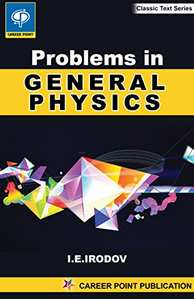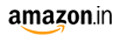# Problem in General Physics (Classic Test Series) JEE MainM.R.P 200/-150.00(25%)
Product Description

This book of problem is intended as a textbook for students at higher educational institute studying advanced course in physics beside because of the great number of simple problem it may be used by students studying a general course in physics

This book contains about 1900 problems with hints for solving the most complicated ones.

For students convenience each chapter opens with a time saving summary of the principal formulas for the relevant area of physics. As as rule the formulas are given without detailed explanation since a students. Starting solving a problem is assumed to know the meaning of the quantities appearing in the formulas .explanatory notes are only given in that case when misunderstanding may arise.

All the formulas in the text and answer are in SI system. Except in part six, where the Gaussian system is used Quantities data and answer are presented in accordance with the rule of approximation and numerical accuracy. The main Physical Constant and tables are summarized at the end of the book.

The periodic system of elements is printed at the front end sheet and the table of elementary particles at the back sheet of the book.

In the present's edition, some misprint are corrected and a number of problem are substitute by new once or the quantities data in them are changes or refined.